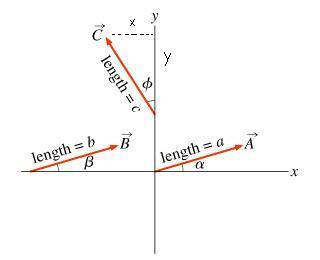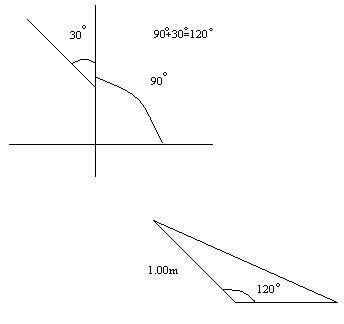# Physic Vector Problem

• Paymemoney

## Homework Statement

Find the components of the vector $$\vec{C}$$ with length c = 1.00 and angle $$\phi = 30.0^{\circ}$$ as shown.## Homework Equations

$$opp=hypcos(\theta)$$
$$adj=hypcos(\theta)$$

## The Attempt at a Solution

tried to use the above equations to answer the question, however when i looked in the answers it was incorrect.
Answer i got was x=0.866 and y=0.5

When i realized that it was between the vector and the y axis, i found that the angle didn't make contact with the x-axis, so my question is What is the angle that $$\vec{C}$$ makes with the positive x axis?

P.S

Hi Paymemoney!## Homework Equations

$$opp=hypcos(\theta)$$
$$adj=hypcos(\theta)$$

erm… they can't both be right, can they?When i realized that it was between the vector and the y axis, i found that the angle didn't make contact with the x-axis, so my question is What is the angle that $$\vec{C}$$ makes with the positive x axis?

Start at the positive x-axis, go round to the y-axis, then go round some more … that's your angle!But the main thing you've done wrong is that the dotted line marked "x" on your diagram isn't x, is it?Hi Paymemoney!erm… they can't both be right, can they?oops, meant to be $$opp=hypsin(\theta)$$

Start at the positive x-axis, go round to the y-axis, then go round some more … that's your angle!But the main thing you've done wrong is that the dotted line marked "x" on your diagram isn't x, is it?ok so i got 600 degrees, what is the next step i should take to solve $$\vec{C}$$

Hi Paymemoney!(have a degree: º and a theta: θ and a phi: φ)
ok so i got 600 degrees …

hold it!

however did you get 600º ?well i went around the the axis 2 times o_o

well i went around the the axis 2 times o_o

Still not 600º.Anyway, just go from the x-axis to the y-axis anticlockwise, and then carry on to C.

ok this is what i have done:tell me if this is correct.

Yes, that's exactly correct.The "first quadrant" (top-right) is 0º to 90º, the "second quadrant" (top-left) is 90º to 180º, and so on.